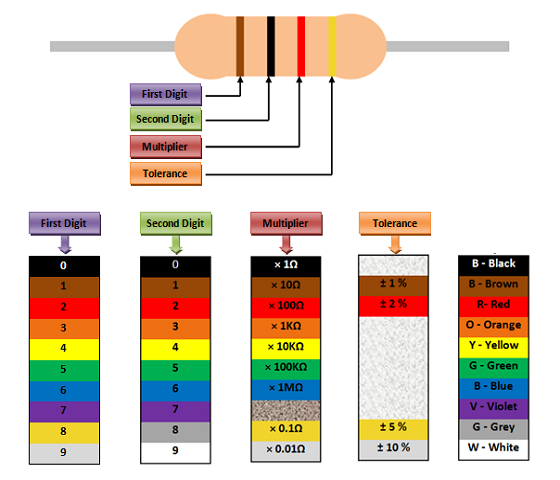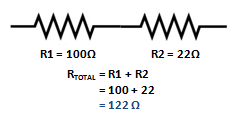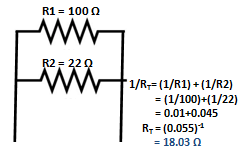# Resistor

[Click the image to enlarge it]

### Pin Configuration

Resistors have two leads, there is no polarity for a resistor and hence can be connected in both directions.

Note: This document refers only to the carbon film resistors, since they are the most widely used ones for all electronic projects.

### Features

• Carbon Film Resistor
• 4-band Resistor
• Resistor value varies based on  selected parameter
• Power rating varies based on selected parameter

Other Resistor based Components: Higher Power Resistor, Potentiometer (Variable Resistor), LDR (Light Dependent Resistor), Thermistor.

### Resistor parameters selection

Ever wondered what about the types of resistors available in market or how to select one for your project read further. Resistors can be classified based on two main parameters. One is their Resistance (R-ohms) itself and the other is its Power (P-Watts) rating.

The value or resistance decides how much opposition it provides to the flow of current. The greater the resistor value the smaller the current will flow. Resistors are not available in all values, there are only few standard values which are most used and they are listed below

Standard Resistor Values: 0Ω, 1Ω, 10Ω, 22Ω, 47Ω, 100Ω, 150Ω, 200Ω, 220Ω, 270Ω, 330Ω, 470Ω, 510Ω, 680Ω, 1KΩ, 2KΩ, 2.2KΩ, 3.3KΩ,  4.7KΩ, 5.1KΩ, 6.8KΩ, 8.2KΩ, 10KΩ, 20KΩ, 33KΩ, 39KΩ, 47KΩ, 51KΩ, 68KΩ, 100KΩ, 220KΩ, 300KΩ, 470KΩ, 680KΩ, 1MΩ.

Resistors are also classified based on how much current it can allow; this is called Power (wattage) rating. The higher the power rating the bigger the resistor gets and it can also more current. A Quarter Watt (1/8) resistor is used by default for all electronics projects. However if you need otherwise, the following are the common options

Standard Power Ratings: 1/4 Watts, 1/8 (Quarter) Watts, ½ (Half) Watt, 1 (One) Watts, 2 (Two) Watts, Higher Watt resistor.

### Identifying a Resistor

In order to identify the resistance value of a Resistor, we have to look at its colour code. Yeah! It would have been easy if the value was directly written, but still with little practise from below we can start reading the resistor values.

As said earlier, Resistors are not available in all values. So if you project needs a particular value which is not commonly available then you have to make up the value by using either series or parallel combination as shown below.Resistor in Series:

The value of resistors get added up when they are placed in series.Resistor in parallel:

The value of resistor gets inversely added up when they are parallel.### Applications

• Current limiting Resistor
• To create voltage drop
• Pull up / Pull down Resistor
• Potential dividers
• To measure current as shunt resistor
Component Datasheet